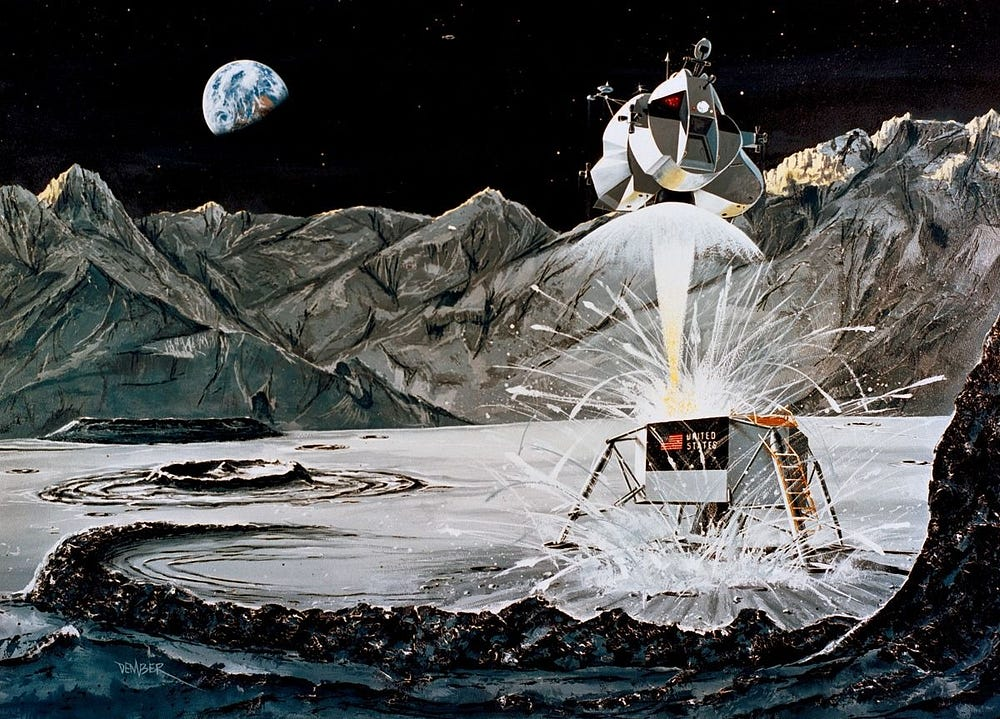# Breaking Down Richard Sutton’s Policy Gradient With PyTorch And Lunar Lander

Source: Deep Learning on Medium## Theory Behind The Policy Gradient Algorithm

Before we can implement the policy gradient algorithm, we should go over specific math involved with the algorithm. The math is very straight-forward and very easy to follow and for the most part, is reinterpreted from the OpenAI resource mentioned above.

First, we define tau to be a trajectory or a sequence of actions and the corresponding rewards obtained by executing these actions.

Now, we can define a function of the rewards to be a discounted or undiscounted (for episodic tasks) sum of trajectory’s rewards. In practice, we will find that even for episodic tasks, it is more beneficial to use discounted sum of rewards, defined as follows:

Second, we define performance measure J as an expected value of some function of the rewards that came from the most recent batch of trajectories (obtained under the current policy execution).

Let’s investigate the performance measure a little closer. By the definition of expectation, we obtain:

It is essential to understand that we would like to use the gradient of the performance measure to optimize our policy (agent). Hence, we obtain the following:

Now, there is an excellent trick or the log-derivative trick that comes from the following identity:

We can use this trick to replace the gradient of the probability of the trajectory with the product of the probability and gradient of the log-probability of the trajectory, or:

If we look closely at the right-hand side, we can notice that it is an expectation itself:

But what is the log-probability of the trajectory? It turns out that we can use the chain rule to define the probability over the trajectory:

Intuitively this chain rule makes a lot of sense. We sample the initial state from some initial state distribution. Then, as our actions are stochastic, we choose an action with some probability over the action space, which is our policy. Finally, the transition model is stochastic too; hence, we multiply by the probability of transitioning from the previous state to the next state. We continue this process until we reach the end of the episode.

Now, let us look into the log-probability distribution over the trajectory space:

Let us calculate the gradient of the log-probability over the trajectory space with respect to the parameters of the policy:

We see that only the policy probability distribution depends on the policy parameters. Hence, the rest of the terms evaluate to zero. Finally, we can put all of the math together to obtain:

Now, since the gradient of the performance measure is an expectation, we can estimate it with sampling, which is extremely easy. We will generate several trajectories under the current policy (as the policy gradient is an on-policy algorithm) and then will calculate the mean of the weighted (by R(tau)) log-probabilities that we obtain from the agent (policy).

## Rewards-To-Go

Instead of the sum of the discounted rewards, we are going to use the sum of the discounted rewards from the time t to the end of the episode. These are called rewards-to-go and are used more frequently in policy gradient methods as the actions that are committed after time t should not have any effect on the rewards that were obtained before these actions happened.

## Entropy Bonus

In the code, we will also use an entropy bonus to discourage strict certainty. The idea is relatively simple: we subtract the entropy of the policy from the “loss” during the policy optimization. If the agent is overly confident in its actions, then the entropy of the policy becomes small, and the bonus vanishes. The entropy of the policy is a recurrent theme in reinforcement learning and is used in other algorithms such as Soft Actor-Critic.

## Baseline

We also are going to use a baseline. A baseline is a quantity that gets subtracted from R(tau) without affecting the expectation because, typically, the baseline is a state-specific quantity. We will use a state-specific mean of the trajectory’s rewards as a baseline.

The baseline reduces variance in the policy gradient estimation. Intuitively, it makes a lot of sense, especially in the case of the CartPole problem. Suppose that our agent can balance the pole for 2 seconds. Is that good or bad? If, on average, the agent balanced the pole for 1 second before this episode, then yes, it is much better performance. The policy gradient will be estimated to be positive in this case, and the agent will take a step in the direction of further improvement.

However, if the agent on average balanced the pole for 3 seconds before the episode, the policy gradient will be estimated to be negative, and we will still make the step in the right direction, away from the parameters that made the agent balance the pole for 2 seconds. If we don’t use the baseline, both 1 second and 3 seconds and 10 seconds episode will result in similar gradient directions; hence, training might take much longer.

It is important to note that for more complicated problems such as LunarLander, the baseline is less intuitive as we have both negative and positive rewards, and the scale of the rewards is different.